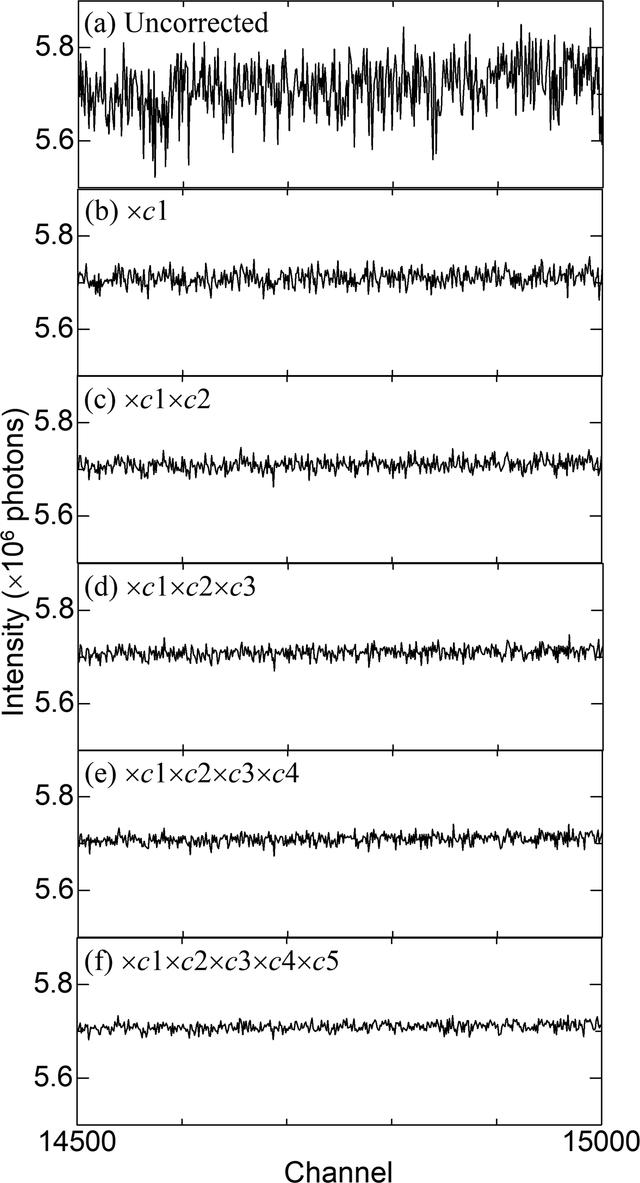disable zoom     view article Figure 4 The scattering data of SiO2 glass from channels 14500 to 15000 at each step in the multi-step process: (a) uncorrected; (b) multiplied by c1 in equation (4); (c) multiplied by c1 in equation (4) and c2 in equation (6); (d) multiplied by c1 in equation (4), c2 in equation (6) and c3 in equation (8); (e) multiplied by c1 in equation (4), c2 in equation (6), c3 in equation (8) and c4 in equation (10); and (f) multiplied by cms in equation (13).JOURNAL OFSYNCHROTRONRADIATION
ISSN: 1600-5775
Volume 26| Part 3| May 2019| Pages 762-773